# 1 Introduction

This tutorial demonstrates some common plot types and associated refinements such as marker selection, titles, coloring, etc. Older versions of SAS relied on text graphics (Proc PLOT) or a suite of procedures in the SAS Graphics package. We now use more flexible and updated options in the ODS Graphics suite. Specifically, the demonstrations below use the SAS procedure SGPLOT which is a general plotting procedure that can generate many different plot types. Further procedures for producing multi-paneled plots or defining custom plot layouts are also available, but are not covered here.

# 2 Data used in the Examples

The data used here describes beak depth and length for two finch species collected on the Gallapagos Islands during two years: Data here. The data was collected by Peter and Rosemary Grant in 1975 and 2012 to examine evolutionary modifications in the finch beaks due to environmental changes. The code to read in and partially print the data is shown below.

``````
/* Read in the Grant finch data. */

data finch_1975;
infile '.\Data\Finch_1975.csv' firstobs=2 delimiter=',';
input Band Species\$ length depth year;
run;

data finch_2012;
infile '.\Data\Finch_2012.csv' firstobs=2 delimiter=',';
input Band Species\$ length depth year;
run;

data all;
set finch_1975 finch_2012;
run;

proc print data=all(obs=12);
run;``````
Obs Band Species length depth year
1 2 fortis 9.4 8.0 1975
2 9 fortis 9.2 8.3 1975
3 12 fortis 9.5 7.5 1975
4 15 fortis 9.5 8.0 1975
5 305 fortis 11.5 9.9 1975
6 307 fortis 11.1 8.6 1975
7 308 fortis 9.9 8.4 1975
8 309 fortis 11.5 9.8 1975
9 311 fortis 10.8 9.2 1975
10 312 fortis 11.3 9.0 1975
11 313 fortis 11.5 9.5 1975
12 314 fortis 11.5 8.9 1975

The data contain information on bird ID (Band), species (fortis and scandens), beak length and depth in mm, and the year of collection (1975 , 2012).

# 3 Common Plot Types

## 3.1 Scatter Plots

The scatter plot is probably the most common plotting method. It is useful for discerning potential relationships and structure between two variables. It displays observations as individual data points on an X-Y set of axes. The initial example below plots beak length and depth against one another using the Scatter statement. The where statement selects out only the 1975 data.

``````
proc sgplot data=all;
where year=1975;
scatter x=length y=depth;
title1 '1975 Finch Data Scatter Plot';
run;
``````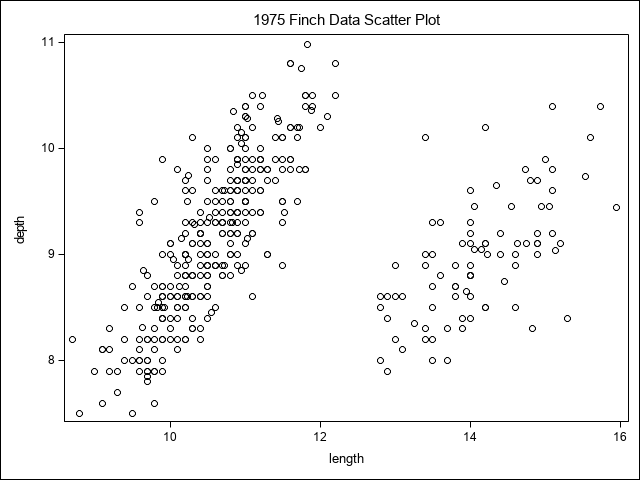In this plot we can see there is a positive relationship between depth and length, but also there are two groupings of data points, indicating some further structure. One aim of plotting is exploration of the data. To examine this structure further, we might opt to see if the groupings are related to some qualitative classification information such as year or species. Since this is only 1975 data, we’ll try species. This is done by adding the group= option to the Scatter statement. We’ve also added a styleattrs statement to specify colors and marker options to control what the data points look like (colored circles with black outlines at a dimension of 10 pixels).

``````
ods graphics;
proc sgplot data=all;
styleattrs datacolors=(green blue);
where year=1975;
scatter x=length y=depth/group=species filledoutlinedmarkers markeroutlineattrs=(color=black) markerattrs=(symbol=circlefilled size=10);
title1 '1975 Finch Data Scatter Plot with Species';
run;
``````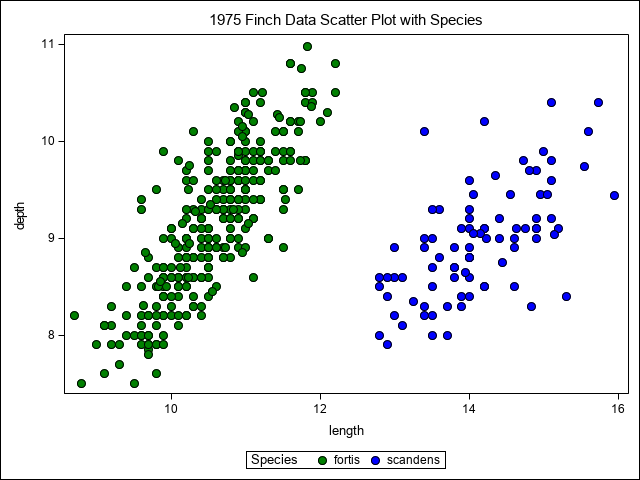We can now see from this simple scatter plot that the groupings are associated with species.

## 3.2 Lines

Another common plot is to plot lines. In the case of regression modeling, we often plot lines along with the scatter plot. In this example the depth variable is fit as a function of length in a linear regression for each species. The output is then saved for plotting. For details on linear regression, see the tutorial on regression.

``````proc sort data=all;
by species year length;
run;

proc reg data=all;
where year=1975;
model depth =length;
by species;
output out=predicted p = pred;
run;
``````

The output data set predicted is then used to do plotting as before with the addition of a SERIES statement which draws lines linking data points. The group= option is used again, this time to create separate lines for each species. The datacontrastcolors= option specifies the colors for the lines.

``````
ods graphics;
proc sgplot data=predicted;
styleattrs  datacolors=(green blue) datacontrastcolors=(green blue);
where year=1975;
scatter x=length y=depth/group=species filledoutlinedmarkers markeroutlineattrs=(color=black) markerattrs=(symbol=circlefilled size=10);
series x=length y=pred/group=species;
title1 '1975 Finch Data Scatter Plot with lines for each Species';
run;
``````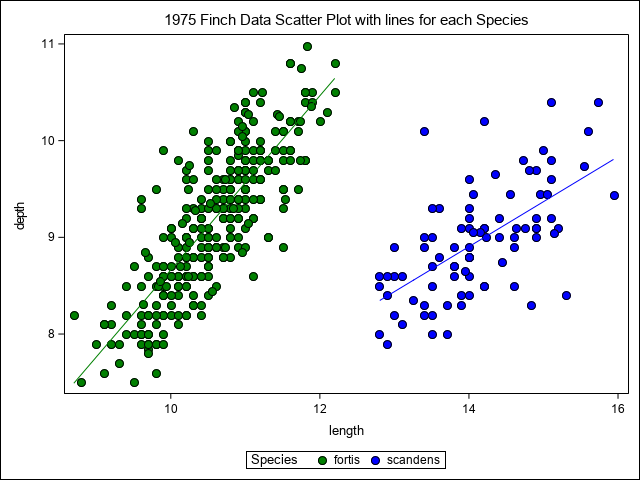## 3.3 Bar Charts

Bar charts are commonly used to display results from ANOVA. In the example below, a MIXED model is used to assess the effects of species and year and their interaction on beak length. The means (LSMEANS) are output and plotted with the vbarparm statement in Proc Sgplot. This statement is used to create vertical bar charts. A similar statement, hbarparm, is available for horizontal bar charts. There are also statements vbar and hbar, but they have fewer options available. The options in the statement also specify upper and lower confidence intervals resulting from the CL option in the lsmeans call from proc mixed. We again use the group option to separate species. This is a clustered bar chart producing a separate set of bars for each year. This is done with the groupdisplay= option.

``````proc mixed data=all;
class species year;
model length = species year species*year;
lsmeans species*year/cl;
ods output LSMeans=means;
run;``````
``````
proc sgplot data=means;
styleattrs datacolors=(green blue) datacontrastcolors=(black black);
vbarparm category=Year response=estimate / LIMITATTRS=(color=black)
limitlower=lower limitupper=upper group=Species OUTLINEATTRS=(color=black) groupdisplay=cluster;
title1 'Finch Data Bar Chart';
run;``````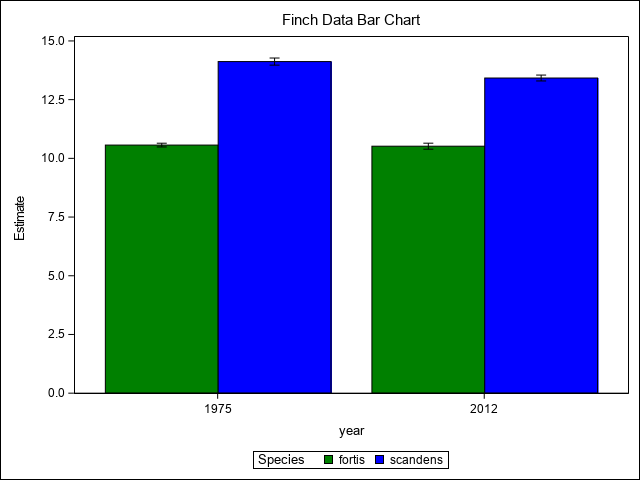## 3.4 Histograms

A histogram displays the frequency distribution of a continuos variable. In Proc Sgplot, the statement histogram is used. The example below looks at the overall distribution of beak lengths.

``````
proc sgplot data=all;
histogram length;
title1 'Histogram of Beak Lengths';
run;``````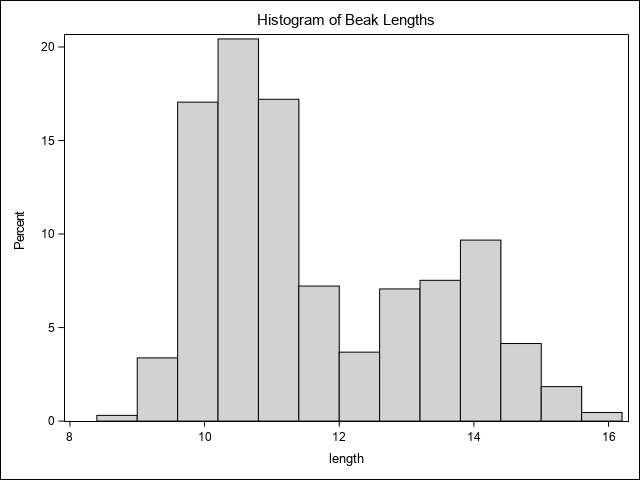Here we can see there are two distinct peaks. As was shown above, these likely relate to species. We can visualize the distribution of each species on one plot by employing the styleattrs statement with two colors as well as the group= option in the histogram statement.

``````
proc sgplot data=all;
styleattrs datacolors=(lightgreen lightblue);
histogram length/group=species;
title1 'Histogram of Beak Lengths with Species';
run;``````